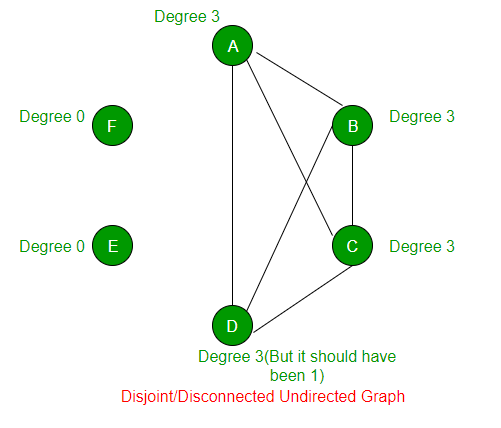# GATE | GATE-CS-2014-(Set-1) | Question 62

An ordered n-tuple (d1, d2, … , dn) with d1 >= d2 >= ⋯ >= dn is called graphic if there exists a simple undirected graph with n vertices having degrees d1, d2, … , dn respectively. Which of the following 6-tuples is NOT graphic?
(A) (1, 1, 1, 1, 1, 1)
(B) (2, 2, 2, 2, 2, 2)
(C) (3, 3, 3, 1, 0, 0)
(D) (3, 2, 1, 1, 1, 0)

Explanation: The required graph is not possible with the given degree set of (3, 3, 3, 1, 0, 0). Using this 6-tuple the graph formed will be a Disjoint undirected graph, where the two vertices of the graph should not be connected to any other vertex ( i.e. degree will be 0 for both the vertices ) of the graph. And for the remaining 4 vertices the graph need to satisfy the degrees of (3, 3, 3, 1).

Let’s see this with the help of a logical structure of the graph :
Let’s say vertices labelled as should have their degree as <3, 3, 3, 1, 0, 0> respectively.Now E and F should not be connected to any vertex in the graph. And A, B, C and D should have their degree as <3, 3, 3, 1> respectively. Now to fulfill the requirement of A, B and C, the node D will never be able to get its degree as 1. It’s degree will also become as 3. This is shown in the above diagram.
Hence tuple <3, 3, 3, 1, 0, 0> is not graphic.

My Personal Notes arrow_drop_up
Article Tags :

Be the First to upvote.

Please write to us at contribute@geeksforgeeks.org to report any issue with the above content.